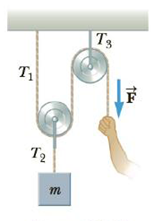Chapter 5, Problem 43P

Chapter
Section
Textbook Problem

The system shown in Figure P5.43 is used to lift an object of mass m = 76.0 kg. A constant downward force of magnitude F is applied to the loose end of the rope such that the hanging object moves upward at constant speed. Neglecting the masses of the rope and pulleys, find (a) the required value of F, (b) the tensions T1, T2, and T3, and (c) the work done by the applied force in raising the object a distance of 1.80 m.Figure P5.43

(a)

To determine
The value of applied force.

Explanation

Given Info:

The mass of the object is 76.0kg .

From the figure of moving pulley,

2T1=T2

Therefore,

Formula to calculate the applied force is,

F=T1=T22=mg2

• m is the mass of object
• g is acceleration due to gravity

Substitute 76.0kg for m and 9

(b)

To determine
The tensions T1 , T2 and T3 .

(c)

To determine
The work done by the applied force.

Still sussing out bartleby?

Check out a sample textbook solution.

See a sample solution

The Solution to Your Study Problems

Bartleby provides explanations to thousands of textbook problems written by our experts, many with advanced degrees!

Get Started